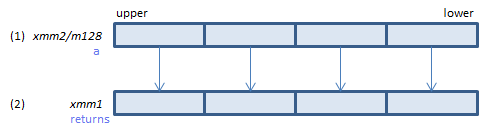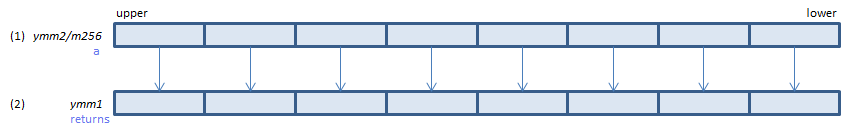## RSQRTPS - Reciprocal SQuare RooT Packed Single

RSQRTPS xmm1, xmm2/m128    (S1
__m128 _mm_rsqrt_ps(__m128 a)
VRSQRTPS xmm1, xmm2/m128    (V1
__m128 _mm_rsqrt_ps(__m128 a)For each float, calculate approximate reciprocal of square root of (1) and set the result to (2). (relative error <= 1.5*2-12)
VRSQRTPS ymm1, ymm2/m256    (V1
__m256 _mm256_rsqrt_ps(__m256 a)For each float, calculate approximate reciprocal of square root of (1) and set the result to (2). (relative error <= 1.5*2-12)
512-bit → VRSQRT14PS / VRSQRT28PS

x86/x64 SIMD Instruction List  Feedback